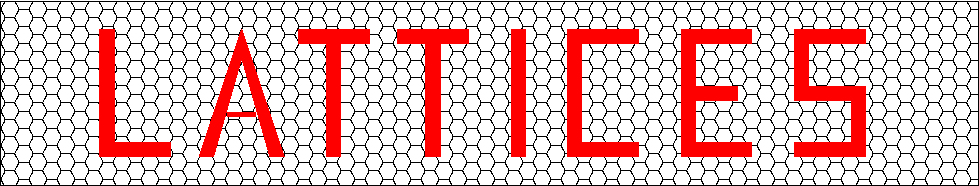## Richard Borcherds' Complete List of Even 25-Dimensional Lattices of Determinant 2

Part of the Catalogue of Lattices, which is a joint project of Gabriele Nebe, RWTH Aachen university (nebe@math.rwth-aachen.de) and Neil J. A. Sloane, AT&T Labs-Research (njasloane@gmail.com).## The Complete List of Even 25-Dimensional Lattices of Determinant 2

Richard Borcherds
Department of Pure Mathematics and Mathematical Statistics
University of Cambridge
Cambridge, England

Taken from R. E. Borcherds, The Leech Lattice and Other Lattices, Ph. D. Dissertation, University of Cambridge, 1984.

• This is a table of the 121 even 25-dimensional lattices of determinant 2.

### Table -2.  The norm -2 vectors of II25,1.

The following sets are in natural 1:1 correspondence:

(1)
Orbits of norm -2 vectors in II25,1 under Aut(II25,1).
(2)
Orbits of norm -2 vectors u of D under Aut(D).
(3)
25 dimensional even bimodular lattices L.
L is isomorphic to u^. Table -2 lists the 121 elements of any of these three sets.

The height t is the height of the norm -2 vector u of D, in other words -(u,w) where w is the Weyl vector of D. The letter after the height is just a name to distinguish vectors of the same height, and is the letter referred to in the column headed ``Norm -2's'' of table -4. An asterisk after the letter means that the vector u is of type 1, in other words the lattice L is the sum of a Niemeier lattice and a1.

The column ``Roots'' gives the Dynkin diagram of the norm 2 vectors of L arranged into orbits under Aut(L). ``Group'' is the order of the subgroup of Aut(D) fixing u. The group Aut(L) is a split extension R.G where R is the Weyl group of the Dynkin diagram and G is isomorphic to the subgroup of Aut(D) fixing u.

``S'' is the maximal number of pairwise orthogonal roots of L.

The column headed ``Norm 0 vectors'' desribes the norm 0 vectors z corresponding to each orbit of roots of u^ where u is in D, as in 3.5.2. A capital letter indicates that the corresponding norm 0 vector is twice a primitive vector, otherwise the norm 0 vector is primitive. x stands for a norm 0 vector of type the Leech lattice. Otherwise the letter a, d, or e is the first letter of the Dynkin diagram of the norm 0 vector, and its height is given by height(u)-h+1 where h is the Coxeter number of the component of the Dynkin diagram of u.

For example, the norm -2 vector of type 23a has 3 components in its root system, of Coxeter numbers 12, 12, and 6, and the letters are e, a, and d, so the corresponding norm 0 vectors have Coxeter numbers 12, 12, and 18 and hence are norm 0 vectors with Dynkin diagrams E64, A11D7E6, and D10E72.